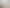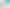# Conversion Chart

2

#### By Pat Duran@kitchenChatter

I hope this helps you all out.
This is from kitchen Vault and the Graceful Cuisine.

So be a perfectionist, have a look at the precise table for conversions from imperial (USA) to metric.
All are rounded to the nearest whole number.

Rating:

★★★★★ 4 votes

Comments:
Serves:
all

## Ingredients

• #### BY WEIGHT OUNCES BY GRAM (G) AND LIQUID (ML)

• 0.5 oz
=14 g or 15ml
• 1 oz
=28g or 30ml
• 2 oz
=58g or 60ml
• 3 oz
=86g or 85ml
• 4 oz
=114g or 115ml
• 5 oz
=142g or 140ml
• 6 oz
=170g or 170ml
• 7 oz
=198g or 200ml
• 8 oz
=226g or 230ml
• 9 oz
=256g or 260ml
• 10 oz
=284g or 285ml
• 11 oz
=312g or 310ml
• 12 oz
=340g or 340ml
• 13 oz
=368g or 370ml
• 14 oz
=396g
• 15 oz
=426g
• 16 oz
=454g
• 24 oz
=680g

• 1/8 tsp
=0.5ml
• 1/4 tsp
=1ml
• 1/2 tsp
=2.5ml
• 3/4 tsp
=4ml
• 1 tsp
=5ml
• 1 Tbsp
=15ml
• 1 1/2 Tbsp
=25ml
• 1/8 c
=30ml
• 1/4 c
=60ml
• 1/3 c
=80ml
• 1/2 c
=120ml
• 2/3 c
=160ml
• 3/4 c
=180ml
• 1 c
=240ml

• 150^F
=65^c
• 175^F
=80^c
• 200^F
=95^c
• 225^F
=110^c
• 250^F
=120^c
• 275^F
=135^c
• 300^F
=150^c
• 325^F
=160^c
• 350^F
=175^c
• 400^F
=205^c
• 425^F
=220^c
• 450^F
=230^c
• 475^F
=245^c
• 500^F
=260^c

• 1/8 in.
=3mm
• 1/4 in.
=6mm
• 1 in.
=2.5cm
• 1 1/4 in.
=3cm
• 2 in.
=5cm
• 6 in.
=15cm
• 8 in.
=20cm
• 9 in.
=22.5cm
• 10 in.
=25cm
• 11 in.
=28cm
• 12 in.
=30cm
• 13 in.
=32.5cm

## How to Make Conversion Chart

1. If you want to do the math yourself - here is the formula:
Multiply the number of ounces by 0.125 which is the number of cups per ounce.
For example 16 oz.x 0.125=2 cups
2. Divide the value in grams by 28, which is the number of grams per ounce, to find the number of ounces.
For example 168 grams divide by 28= about 6 oz.
6 oz. x 0.125=0.75 cups or 3/4 cup.

## About Conversion Chart

Course/Dish: Other Non-Edibles
Other Tag: Quick & Easy

## You May Also Like:### pinch tips: How to Keep Knives Sharp Like a Pro

Kitchen Crew @JustaPinch

Thanks to these tips from the Just A Pinch Test Kitchen your knives will always be sharp!### 7 Tips for Black Friday Shopping

Kitchen Crew @JustaPinch

If you love a good bargain, chances are, you love Black Friday. The day after Thanksgiving is widely known as the nation’s single biggest shopping day all year long. True,...### 13 Time-Saving Kitchen Gadgets

Kitchen Crew @JustaPinch

Everyone needs a few kitchen shortcuts. We've gathered 13 items that will save you time in the kitchen, and maybe your sanity too. Herb Scissors Saves space and time......